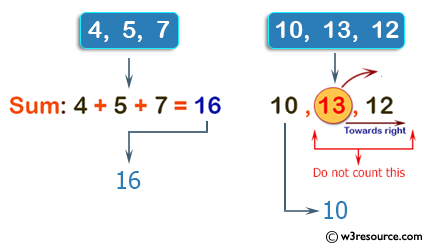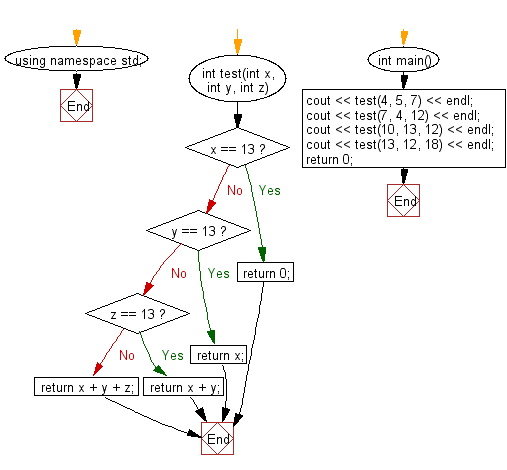﻿ C++ : Sum 3 integers, if one is 13, exclude it in total# C++ Exercises: Compute the sum of the three integers. If one of the values is 13 then do not count it and its right towards the sum

## C++ Basic Algorithm: Exercise-53 with Solution

Write a C++ program to compute the sum of the three integers. If one of the values is 13 then do not count it and its right towards the sum.

Sample Solution:

C++ Code :

``````#include <iostream>

using namespace std;

int test(int x, int y, int z)
{
if (x == 13) return 0;
if (y == 13) return x;
if (z == 13) return x + y;
return x + y + z;
}

int main()
{
cout << test(4, 5, 7) << endl;
cout << test(7, 4, 12) << endl;
cout << test(10, 13, 12) << endl;
cout << test(13, 12, 18) << endl;
return 0;
}
``````

Sample Output:

```16
23
10
0
```

Pictorial Presentation:Flowchart:C++ Code Editor: